# Images and NumPy in Computer Vision

## Images and NumPy in Computer Vision

In this article, I am going to discuss Images and NumPy in Computer Vision. Please read our previous article where we discussed Transfer learning using Keras.

#### NumPy Arrays in Computer Vision

NumPy arrays are a fundamental part of the Python scientific computing ecosystem. They provide a fast and efficient way to perform numerical computations on large datasets. In this article, we will explore the basics of NumPy arrays and some of the operations that can be performed on them.

A NumPy array is a multidimensional array object that can hold values of a single data type. NumPy arrays are homogeneous, which means that all elements in the array must be of the same data type. They are more efficient than Python lists because they are stored in contiguous memory locations, making it easy to perform mathematical operations on the entire array.

To create a NumPy array, you can use the numpy.array() function. This function takes a Python list as input and returns a NumPy array. Here’s an example:

```import numpy as np

my_list = [1, 2, 3, 4, 5]
my_array = np.array(my_list)
print(my_array)
```
###### Output: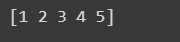You can also create NumPy arrays filled with zeros or ones using the numpy.zeros() and numpy.ones() functions, respectively. Here’s an example:

```import numpy as np

zeros_array = np.zeros(5)
print(zeros_array)

ones_array = np.ones(5)
print(ones_array)
```
###### Output: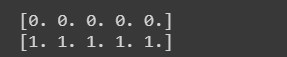NumPy arrays can be multidimensional. You can create a two-dimensional array by passing a list of lists to the numpy.array() function. Here’s an example:

```import numpy as np

my_list = [[1, 2, 3], [4, 5, 6], [7, 8, 9]]
my_array = np.array(my_list)
print(my_array)
```
###### Output:NumPy arrays support a wide variety of mathematical operations. You can perform element-wise addition, subtraction, multiplication, and division using the +, -, *, and / operators, respectively. Here’s an example:

```import numpy as np

a = np.array([1, 2, 3])
b = np.array([4, 5, 6])

print(a + b)
print(a - b)
print(a * b)
print(a / b)
```
###### Output: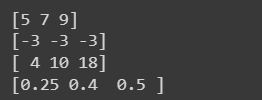You can also perform mathematical operations between a NumPy array and a scalar value. Here’s an example:

```import numpy as np

a = np.array([1, 2, 3])

print(a + 1)
print(a - 1)
print(a * 2)
print(a / 2)
```
###### Output: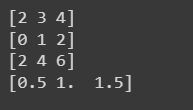NumPy arrays also support Boolean operations. You can use the ==, !=, <, >, <=, and >= operators to perform element-wise comparisons between two NumPy arrays. Here’s an example:

```import numpy as np

a = np.array([1, 2, 3])
b = np.array([2, 2, 3])
print(a==b)
```
###### Output: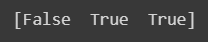#### What is an Image?

In the context of a computer, an image refers to a two-dimensional array of pixels that represent a visual representation of an object or scene. Images can be either static or dynamic and can be captured from a variety of sources, such as cameras, scanners, or generated by computer software.

The term “pixel” is short for “picture element”. Each pixel represents a single point in an image and contains information about its color and intensity. The number of pixels in an image determines its resolution. Higher-resolution images have more pixels per unit of area, resulting in a sharper, more detailed image.

Images can be stored in various file formats, such as JPEG, PNG, or BMP. Each file format uses a different method of compressing and storing image data, which can impact the quality and file size of the image.

One of the most important aspects of working with images on a computer is the ability to manipulate and process them. Image processing uses mathematical algorithms and techniques to enhance or modify images. This can include operations such as resizing, cropping, filtering, and noise reduction.

Computer vision is a field of study that involves the use of computers to analyze and interpret images. Computer vision algorithms can be used to perform tasks such as object recognition, face detection, and image classification. This has many practical applications, such as self-driving cars, medical imaging, and security systems.

In addition to 2D images, computers can also work with 3D images, which represent a visual representation of an object in three dimensions. 3D images are commonly used in fields such as architecture, engineering, and video game development.

Overall, images play an important role in many areas of computing, from basic image manipulation to more advanced applications such as computer vision and 3D modeling.

##### Images and NumPy

Images are a fundamental part of our visual experience and are widely used in many fields, including photography, design, and science. To manipulate and analyze images, computers rely on numerical arrays, and one of the most popular libraries for handling numerical arrays in Python is NumPy.

NumPy is a powerful library that provides tools for working with large, multi-dimensional arrays and matrices. It is widely used in scientific computing, data analysis, and machine learning, and is particularly useful for handling images.

In NumPy, images can be represented as multi-dimensional arrays, where each element in the array represents a pixel in the image. For example, a grayscale image can be represented as a two-dimensional array, where each element corresponds to the intensity of a pixel in the image. The intensity values can range from 0 to 255, where 0 represents black and 255 represents white. Here’s an example of how to create a NumPy array from an image file using the Pillow library:

```from PIL import Image
import numpy as np

# Open the image and convert it to grayscale
img = Image.open('example_image.jpg').convert('L')

# Convert the image to a NumPy array
img_array = np.array(img)
```

In this example, we first open an image file using the Pillow library and convert it to grayscale using the convert() method. We then convert the grayscale image to a NumPy array using the np.array() function.

Once an image is represented as a NumPy array, we can perform a wide range of operations on it. For example, we can resize the image using the resize() function, we can apply filters such as blurring or sharpening using functions from the SciPy library, or we can even perform advanced computer vision techniques such as object detection or segmentation using libraries such as OpenCV. Here’s an example of how to resize an image using NumPy:

```import numpy as np
from PIL import Image

# Open the image and convert it to grayscale
img = Image.open('example_image.jpg').convert('L')

# Convert the image to a NumPy array
img_array = np.array(img)

# Resize the image
resized_array = np.resize(img_array, (200, 200))

# Convert the resized array back to an image and save it
resized_img = Image.fromarray(resized_array)
resized_img.save('resized_image.jpg')
```

In this example, we first create a NumPy array from an image file as we did in the previous example. We then resize the image to a size of 100×100 pixels using the np.resize() function. Finally, we convert the resized NumPy array back to an image using the Image.fromarray() function and save it to a file.

In conclusion, NumPy is a powerful library that provides tools for working with numerical arrays, including images. By representing images as NumPy arrays, we can perform a wide range of operations on them, from basic manipulations such as resizing and filtering to advanced computer vision techniques.